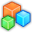# Microsoft Small BasicProgram Listing: LZL364
'Initialise graphics window
GraphicsWindow.Hide()
gw = 800
gh = 600
GraphicsWindow.CanResize = "False"
GraphicsWindow.Top = (Desktop.Height-gh)/2
GraphicsWindow.Left = (Desktop.Width-gw)/2
GraphicsWindow.Title = "Fruit Machine 2"
GraphicsWindow.Width = gw
GraphicsWindow.Height = gh
GraphicsWindow.BackgroundColor = "LightBlue"
Rand1 = 0
i = 0
'Show window
GraphicsWindow.Show()

gw = gw-200
GraphicsWindow.MouseDown = OnMouseDown

delay1=500

GraphicsWindow.PenColor = "Black"
GraphicsWindow.DrawLine(gw,0,gw,gh)

GraphicsWindow.BrushColor = "Black"
GraphicsWindow.DrawBoundText(gw+15,580,50,"RESPIN")
GraphicsWindow.DrawBoundText(gw+115,580,50,"QUIT")'Label

Spinrolls()

'MAIN LOOP ( ui thread) DO ALL SCREEN DRAWS AND USSUALLY PROCESSING HERE
While "True"
If mouseDown Then
Spinrolls()
mouseDown = "False"
EndIf

Program.Delay(15)
EndWhile

Sub Spinrolls

Rand1 = 0
i = 0
For i = 0 To 10 'Rand1

GraphicsWindow.BrushColor = "Red"
GraphicsWindow.DrawBoundText(gw+115,220,70,"Rand1")
GraphicsWindow.DrawText(gw+115,240,Rand1)
Rand1 = Rand1+1
GraphicsWindow.DrawBoundText(gw+115,320,70,"i")
GraphicsWindow.DrawText(gw+115,340,i)

Program.Delay(delay1)
If i < 10 Then
'Clear contents of i and Rand1 until i=10
GraphicsWindow.BrushColor = "LightBlue"
GraphicsWindow.FillRectangle(gw+115,240,70,20)
GraphicsWindow.FillRectangle(gw+115,340,70,20)
EndIf
EndFor
EndSub

Sub OnMouseDown 'THIS AN EVENT HANDLER. USSUALLY JUST SET A FLAG e.g. mouseDown = "true"
'the flag will be read by the Main Loop

xm = GraphicsWindow.MouseX
ym = GraphicsWindow.MouseY

'Respin button
If (xm > gw+15 And xm < gw+85) Then
If (ym > 580 And ym < 595) Then

mouseDown = "True"
'Spinrolls() 'Calling this in the Main loop aka UI thread

' GraphicsWindow.BrushColor = "Blue"
' GraphicsWindow.DrawBoundText(gw+115,50,70,"Start1")
'Rand1 = 0
'i = 0
'delay1=500 ' delay timer
'Stop1 = 0
'Spinrolls()

EndIf
EndIf
'Stop button
If (xm > gw+115 And xm < gw+185) Then
If (ym > 580 And ym < 595) Then
iend = "True"
EndIf
If (iend) Then
Program.End()
EndIf
EndIf

EndSub